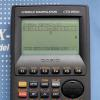# Electron Position With The Atomic Time [Bohr Model] [Fx-3650Pii]

No replies to this topic

### #1frankmar98

frankmar98

Casio Freak

•• Moderator
•• 127 posts
• Gender:Male
• Location:Spain
• Interests:Science, programming

• Calculators:
CFX-9970G
Graph 90+E (fx-GC50)
fx-9860G SD
HP Prime
TI-84+ CE-T
x2 TI-83+
TI-81
fx-4800p, fx-3650PII
fx-991SPX, fx-991ES PLUS, fx-100W

Posted 24 April 2016 - 05:53 PM

```ClrMemory:
?=>C:
?=>D:
5.29E-11*C^2=>B:
(D*2.419E-17*C*6.63E-34)/(2*pi*9.11E-31*B^2)=>A:
B*cos(A)=>X:
B*sin(A)=>Y:
X(output triangle)
Y(output triangle)
```

All physic constants and magnitudes are in the International System of Units, except when you input the time, that is in the atomic unit of time (but I convert it to seconds to be coherent).

Angles are in radians, if the calculator is in degrees, it doesn´t work.

The constansts and formulas used are:

v=S/t

m*v*r=n*(h/2pi)

r_c = (r * cos a, r * sin a)

Bohr´s first atomic radius = 5.29E-11 m

Mass of the electron = 9.11E-31 kg

Planck´s constant = 6.63E-34 J*s

1 second = 2.419E+17 Atomic Time Units

The results are displayed in meters, and the (0,0) point is the nucleous of the atom.

Variables:

C: Atomic Bohr´s level (of the electron that we want to study) (adimensional)

D: Time (ATU)

A: Angle of the electron (considering that when time is 0, angle is 0) (rad)

X and Y: Electron positon (meters)

Regards!

P.D: I´ve edited it to complete it

Edited by frankmar98, 25 April 2016 - 12:58 PM.

#### 0 user(s) are reading this topic

0 members, 0 guests, 0 anonymous users